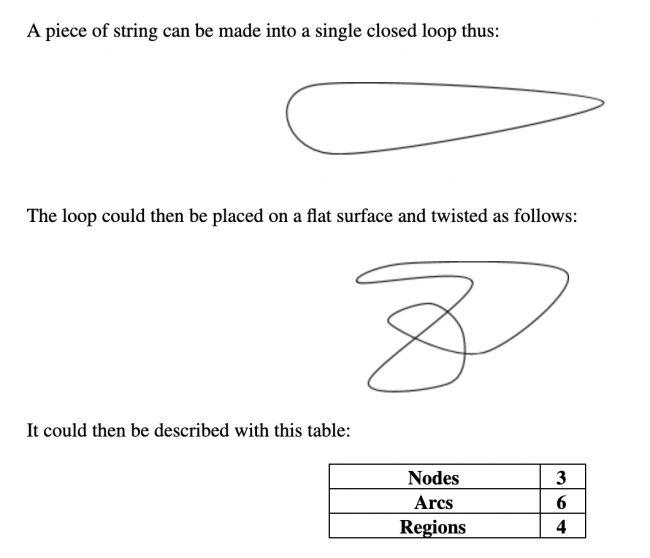# Twisted Loops

Preview:Students investigate the relationship between nodes, arcs and regions for any twisted loop of string.

Project
Big Idea:
Grade 8: Number represents, describes, and compares the quantities of ratios, rates, and percents.
Curricular Competency:
Reasoning and analyzing: Use reasoning to explore and make connections
Understanding and solving: Develop, demonstrate, and apply mathematical understanding through play, inquiry, and problem solving
Understanding and solving: Visualize to explore mathematical concepts
Communicating and representing: Communicate mathematical thinking in many ways
Communicating and representing: Explain and justify mathematical ideas and decisions